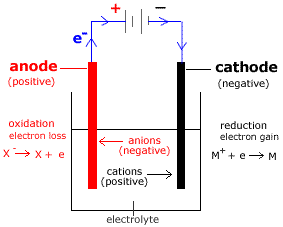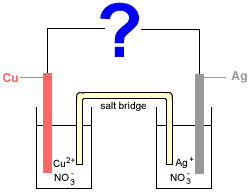# Electrolysis and Electrolytic Cells Concepts Chemistry Tutorial

## Key Concepts

• Electrolysis is the process in which an electric current is used to bring about an electrochemical reaction which does not occur spontaneously (Eo for the electrolytic cell is negative1).
• Electrolytic Cells convert electrical energy into chemical energy.
• Applied voltage (emf) must be greater than the emf for the spontaneous redox reaction, ie greater than Eo.
• An electrolytic cell as shown below must have:(a) An electrolyte containing the species that will undergo electrolysis

(b) 2 Electrodes : conductors used to permit the flow of electrons in an electrochemical cell and provide surfaces at which the reactions take place.
One electrode is the anode, the other is the cathode.

(c) A power supply (a battery or power pack in the school laboratory).

(d) Wires or leads connecting the electrodes to the power supply.

• Within the electrolytic cell:

Electrode
name
Electrode
polarity
Reaction Ion
migration
Observation
anode positive
(+)
oxidation
at anode
negative ions migrate to anode anode disintegrates
cathode negative
(-)
reduction
at anode
positive ions migrate to cathode solid deposits

• Electrons flow from anode to cathode (electrons flow from positive to negative).
• If more than one reaction is possible, the reaction with the lowest Eo will occur.
• Mass of substance produced electrolytically is proportional to the quantity of electricity flowing.

No ads = no money for us = no free stuff for you!

## Electrolytic Cell

A supply of electricity such as a battery or a power pack in the school laboratory is required to drive the electrolytic cell reactions.

Consider the electrolytic cell shown below for the electrolysis of MX:• The electrolyte contains MX (made up of M+ cations and X- anions).
• Anode is positive (+).
Oxidation (loss of electrons) occurs at the anode.
• Cathode is negative (-).
Reduction (gain of electrons) occurs at the cathode.
• Anions (X-) flow towards the anode (positive electrode).
• Cations (M+) flow towards the cathode (negative electrode).
• Electrons flow from the anode (+) to the cathode (-) through the wires connecting the electrodes to the power supply.

The reactions occurring in this electrolytic cell can be represented as shown below:

Electrolytic Cell Reactions
anode:
(oxidation)
X- X + e-     Eo1
cathode:
(reduction)
M+ + e- M     Eo2

cell: X- + M+ X + M Eocell = -ve

Note: Eocell = Eo1 + Eo1 = negative

EMF (volts) required to drive this non-spontaneous reaction is greater than Eocell for the spontaneous redox reaction.

Do you know this?

Play the game now!

## Comparison of Galvanic (Voltaic) Cells and Electrolytic Cells

Consider the following electrochemical cell made up of two half-cells connected by a salt bridge:

• Half-Cell 1: a solid copper electrode (Cu) sits in an aqueous solution of copper(II) nitrate (Cu(NO3)2(aq))
• Half-Cell 2: a solid silver electrode (Ag) sits in an aqueous solution of silver nitrate (AgNO3(aq))The relevant half-cell equations obtained from a table of standard reduction potentials are:

 Cu2+ + 2e- → Cu(s) Eo = +0.34 V Ag+ + e- → Ag(s) Eo = +0.80 V

Whether copper (Cu) is oxidised to copper ions (Cu2+), or, copper ions (Cu2+) are reduced to copper (Cu) depends on what the ? in the diagram is.

### If ? = voltmeter, galvanometer, or metal wire

If we replace the ? in the diagram with a voltmeter or galvanometer to complete the circuit the reaction would proceed spontaneously:

 anode (oxidation): Cu(s) → Cu2+ + 2e- Eo = -0.34V cathode (reduction): 2Ag+ + 2e- → 2Ag(s) Eo = +0.80V cell:(redox) Cu(s) + 2Ag+ → Cu2+ + 2Ag(s) Eo = -0.34 + 0.80 Eo= +0.46 V

Electrons would spontaneously flow from the negative copper anode to the positive silver cathode generating electricity which would be measured by the voltmeter or galvanometer.
The copper anode would disintegrate and silver would be deposited on the silver cathode.
This type of electrochemical cell is known as a galvanic or voltaic cell.

### If ? = battery or other electricity supply with emf > 0.46 V

If we replace the ? in the diagram with a battery supplying more than 0.46 V then the battery has greater electron pushing power than the spontaneous reaction in the electrochemical cell, forcing the reactions to go in the opposite direction to those above.

 cathode (reduction): Cu2+ + 2e- → Cu(s) Eo = +0.34 V anode (oxidation): 2Ag(s) → 2Ag+ + 2e- Eo = -0.80 V cell:(redox) Cu2+ + 2Ag(s) → Cu(s) + 2Ag+ Eo = +0.34 + -0.80 Eo = -0.46 V

Electrons are pulled out of the positive silver anode and flow to the negative copper cathode.
This reaction is not generating electricity it is using electricity!
The silver anode disintengrates while copper deposits on the copper cathode.
This type of electrochemical cell is known as an electrolytic cell.

Do you understand this?

Take the test now!

## Some Uses of Electrolysis

Can you apply this?

Join AUS-e-TUTE!

Take the exam now!

1. This discussion assumes that all species are present in their standard states so that the electrode potentials are standard electrode potentials.